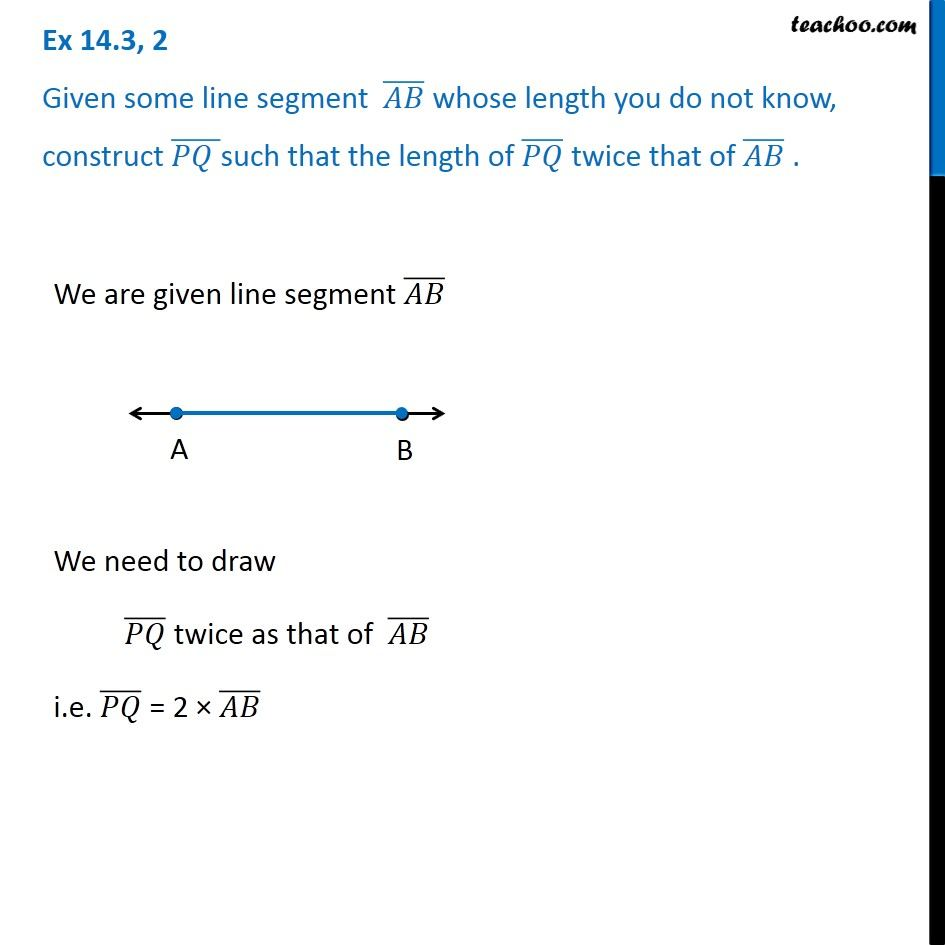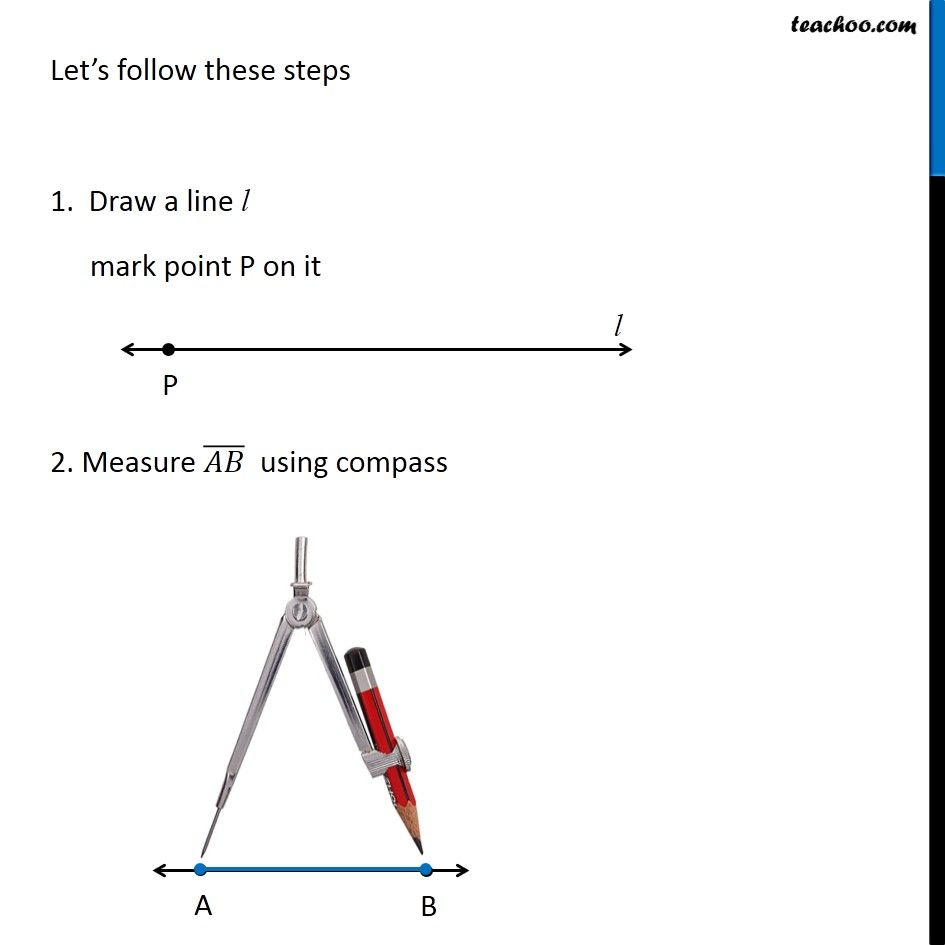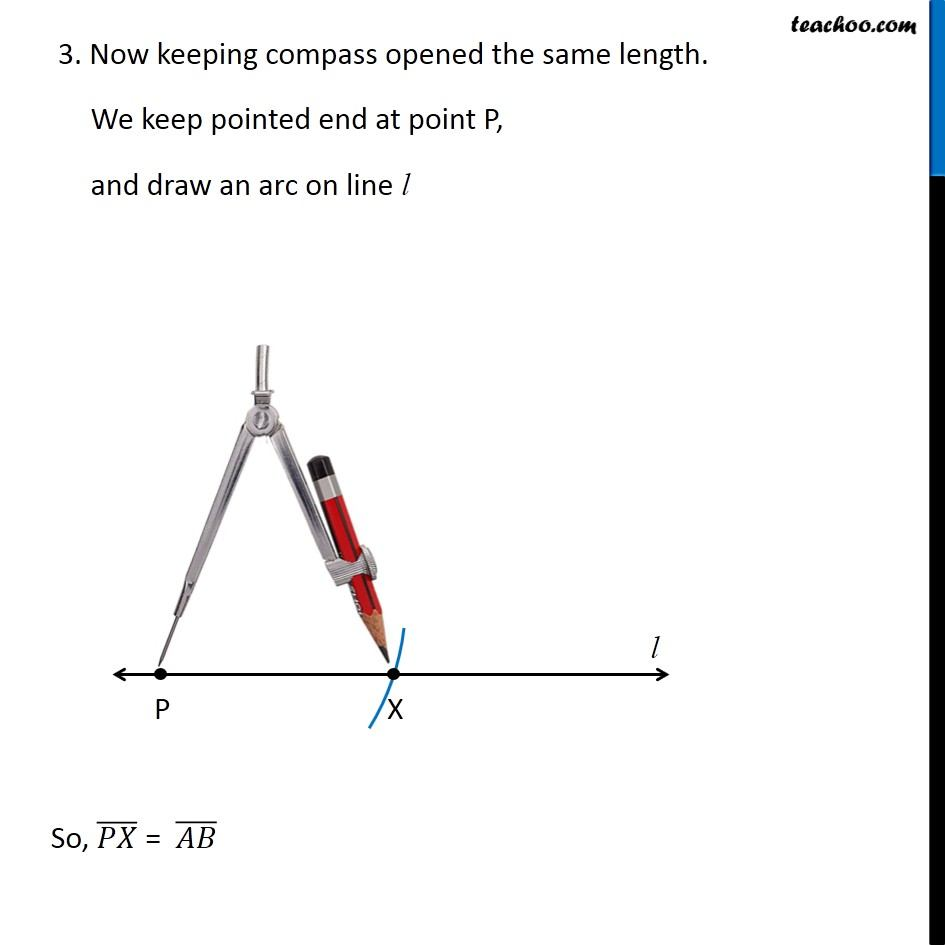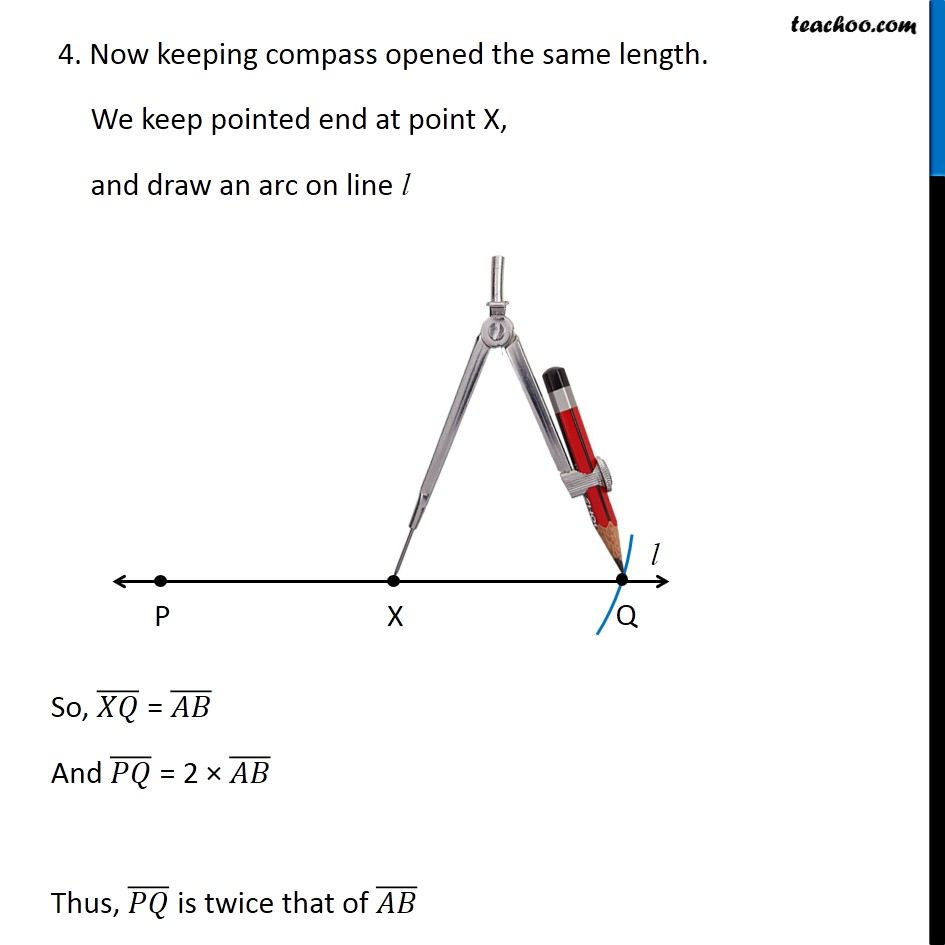Constructing a copy of a given line segment

Practical Geometry
Concept wiseLearn in your speed, with individual attention - Teachoo Maths 1-on-1 Class

### Transcript

Question 2 Given some line segment (𝐴𝐵) ̅ whose length you do not know, construct (𝑃𝑄 ) ̅such that the length of (𝑃𝑄) ̅ twice that of (𝐴𝐵) ̅ . We are given line segment (𝐴𝐵) ̅ We need to draw (𝑃𝑄) ̅ twice as that of (𝐴𝐵) ̅ i.e. (𝑃𝑄) ̅ = 2 × (𝐴𝐵) ̅ Let’s follow these steps 1. Draw a line l mark point P on it 2. Measure (𝐴𝐵) ̅ using compass 3. Now keeping compass opened the same length. We keep pointed end at point P, and draw an arc on line l So, (𝑃𝑋) ̅ = (𝐴𝐵) ̅ 4. Now keeping compass opened the same length. We keep pointed end at point X, and draw an arc on line l So, (𝑋𝑄) ̅ = (𝐴𝐵) ̅ And (𝑃𝑄) ̅ = 2 × (𝐴𝐵) ̅ Thus, (𝑃𝑄) ̅ is twice that of (𝐴𝐵) ̅ 4. Now keeping compass opened the same length. We keep pointed end at point X, and draw an arc on line l So, (𝑋𝑄) ̅ = (𝐴𝐵) ̅ And (𝑃𝑄) ̅ = 2 × (𝐴𝐵) ̅ Thus, (𝑃𝑄) ̅ is twice that of (𝐴𝐵) ̅